# Selina Solutions Concise Maths Class 7 Chapter 3: Fraction (Including Problems) Exercise 3C

Selina Solutions Concise Maths Class 7 Chapter 3 Fraction (Including Problems) Exercise 3C help students understand the various operations on fractions like addition, subtraction, multiplication and division. It also has problems on using ‘of’ and ‘BODMAS’ in the given set of questions. Numerous solved examples are present before the exercise problems to provide a clear understanding among students. Students can self analyse their weaknesses and work on them for a better result in the annual exam. Selina Solutions Concise Maths Class 7 Chapter 3 Fraction (Including Problems) Exercise 3C PDF links are given here.

## Selina Solutions Concise Maths Class 7 Chapter 3: Fraction (Including Problems) Exercise 3C Download PDF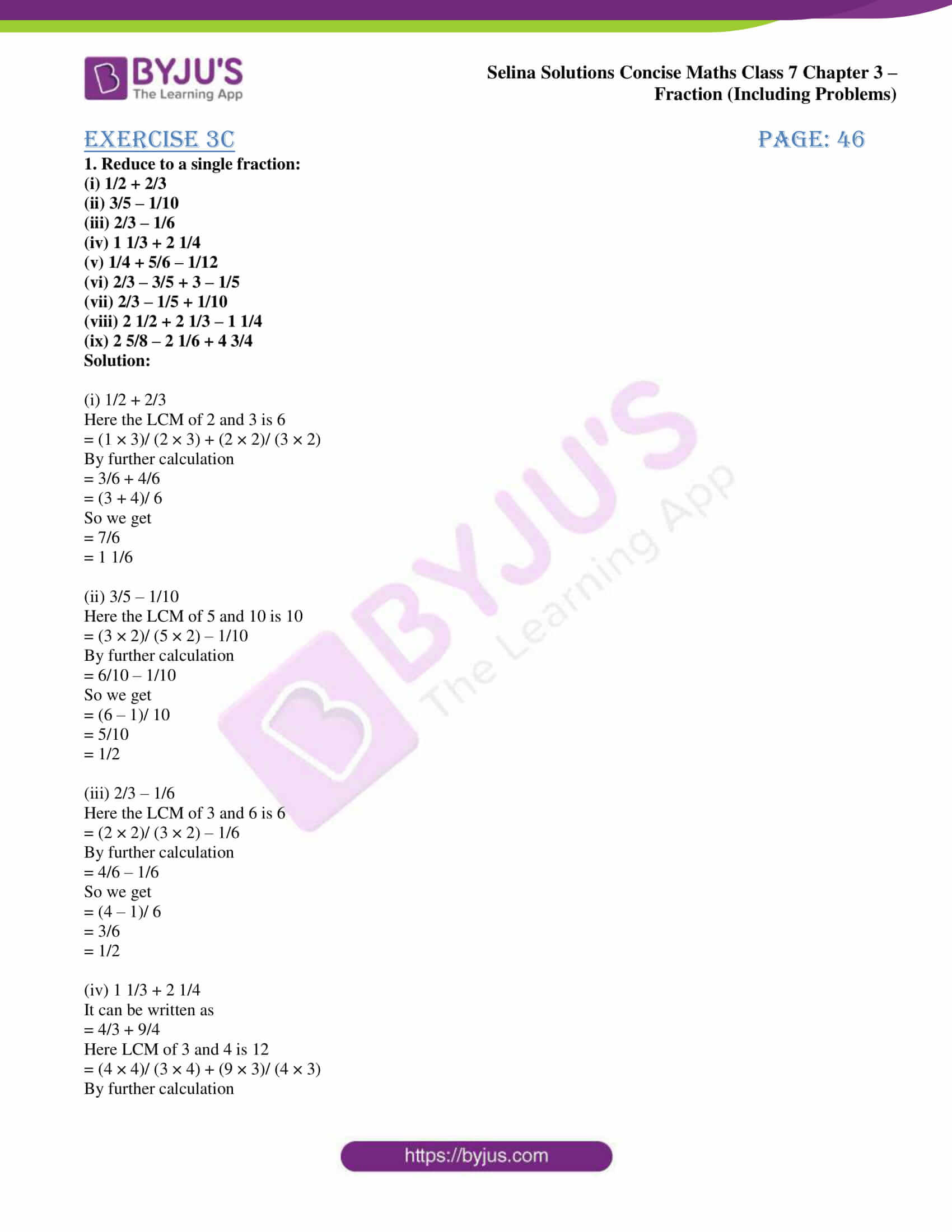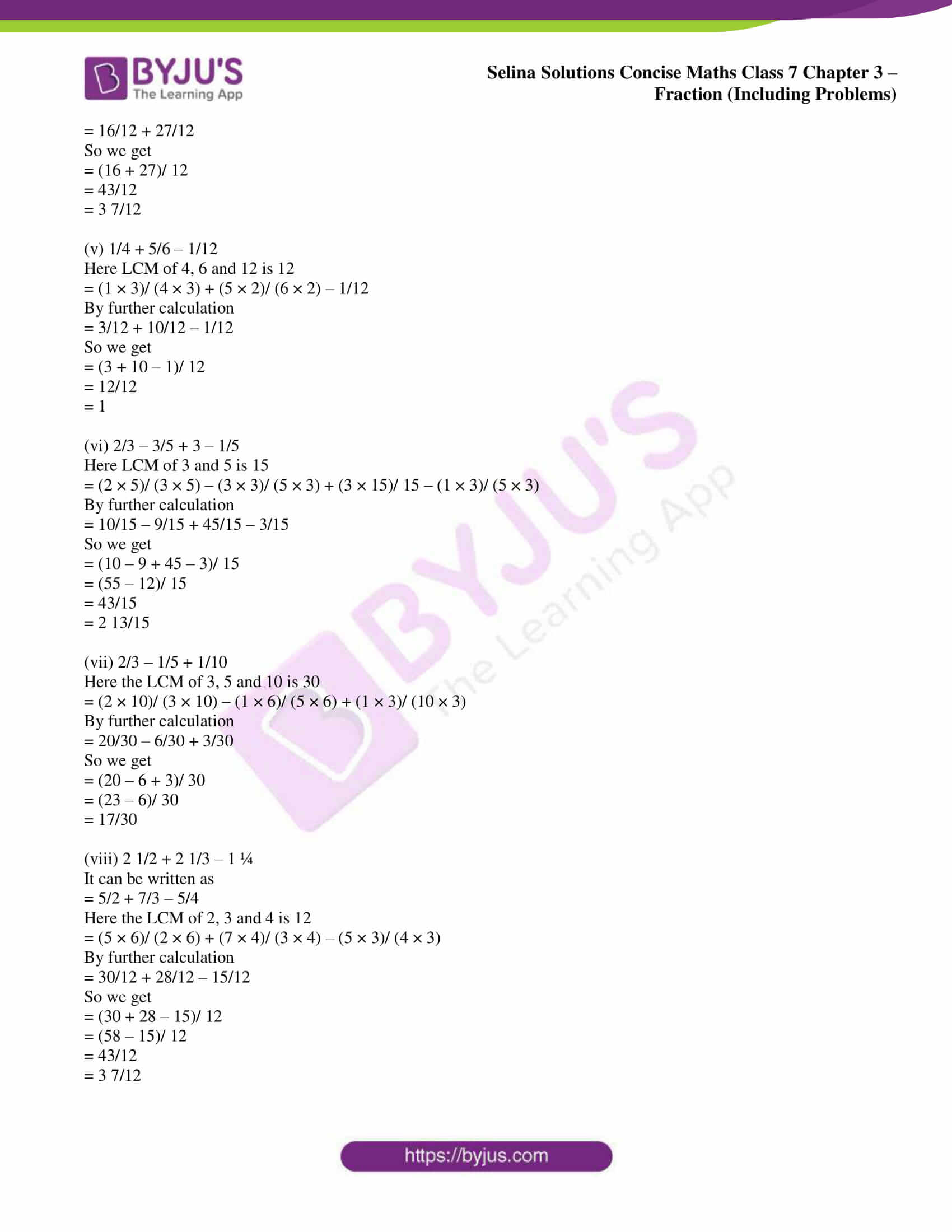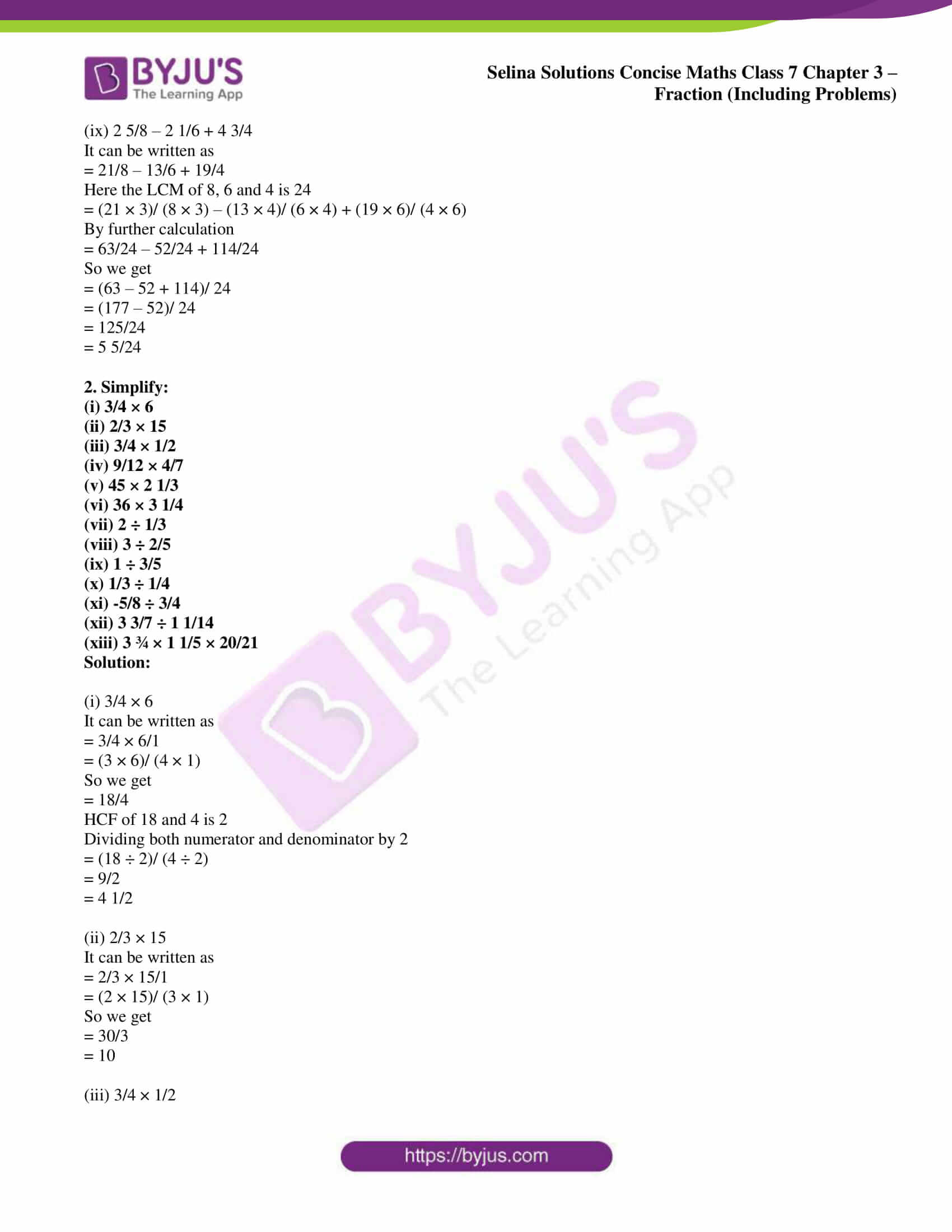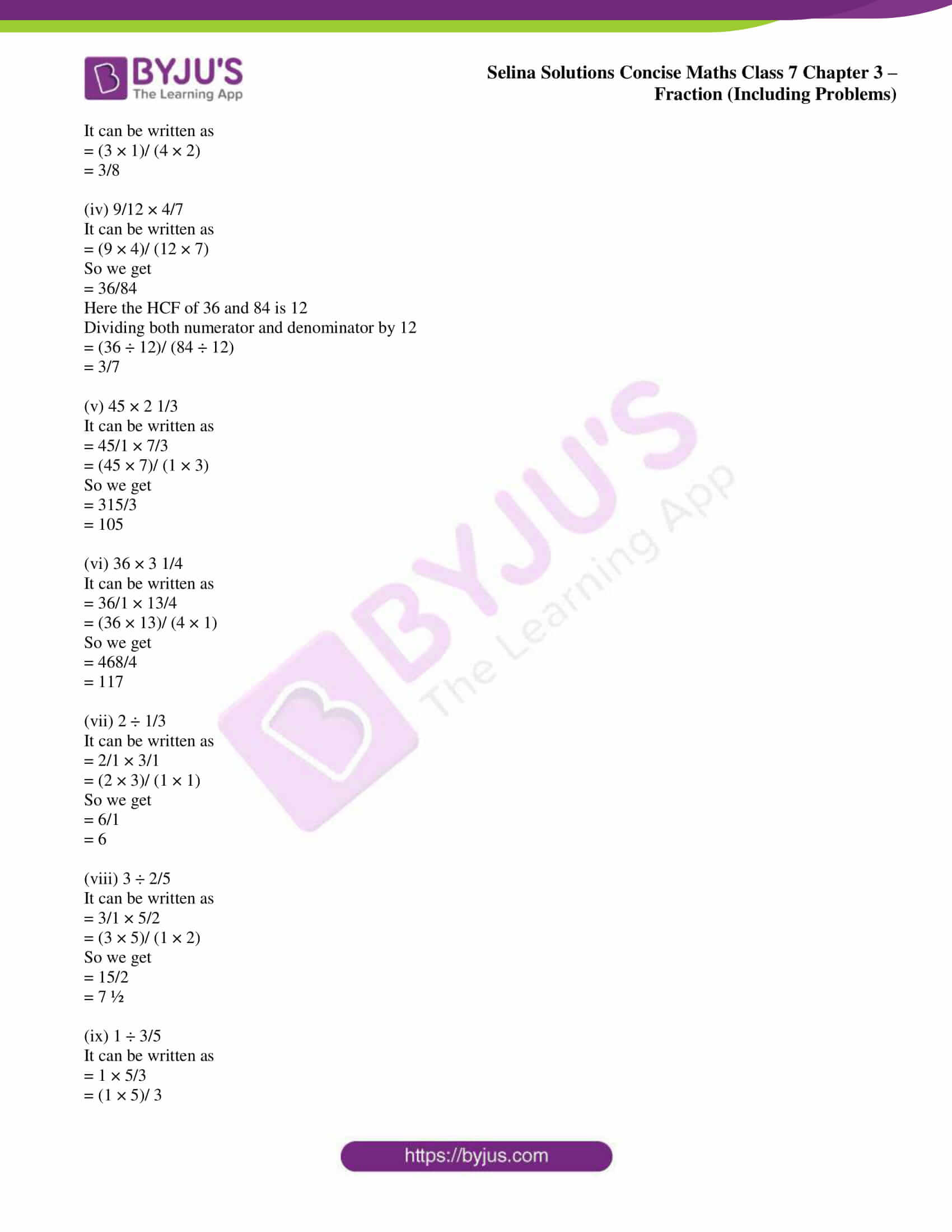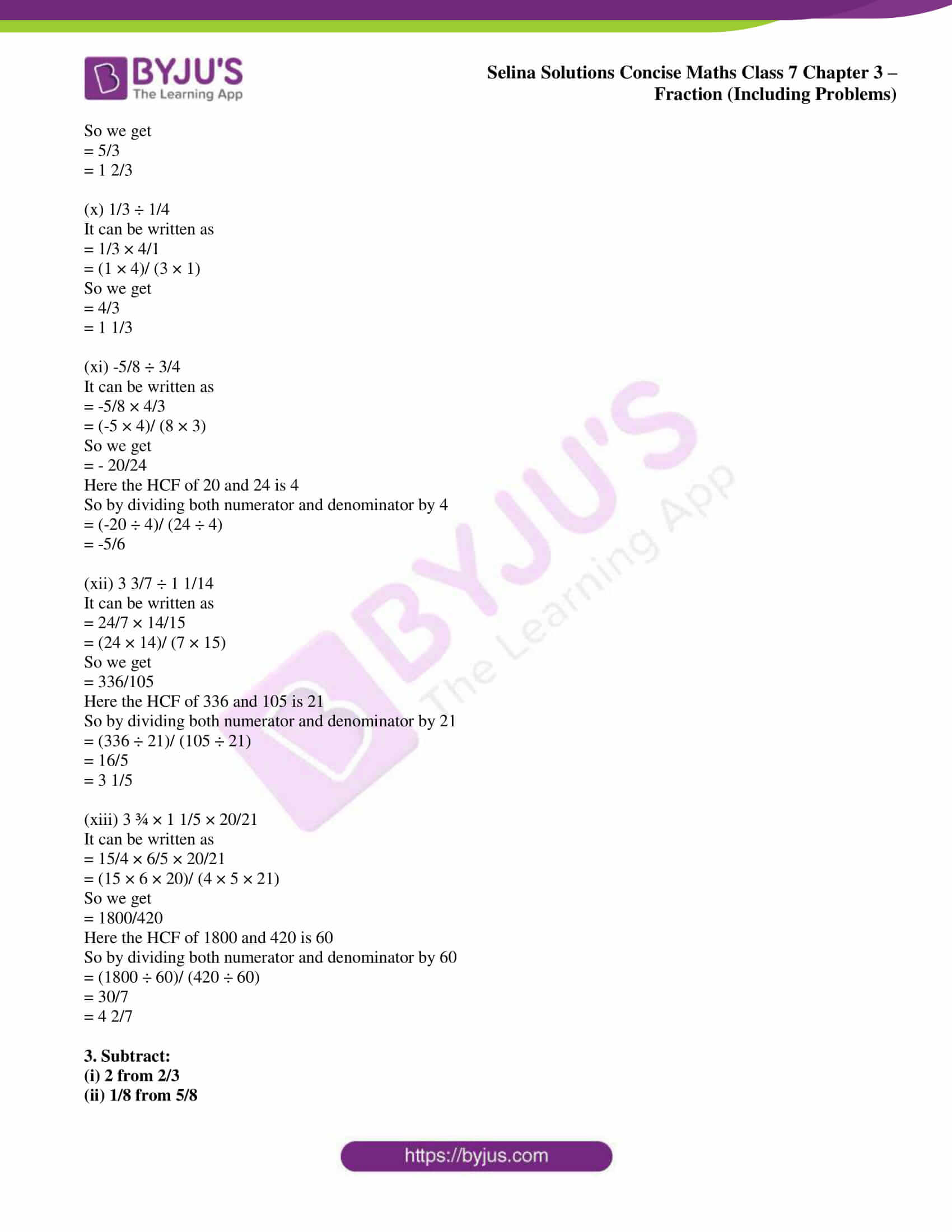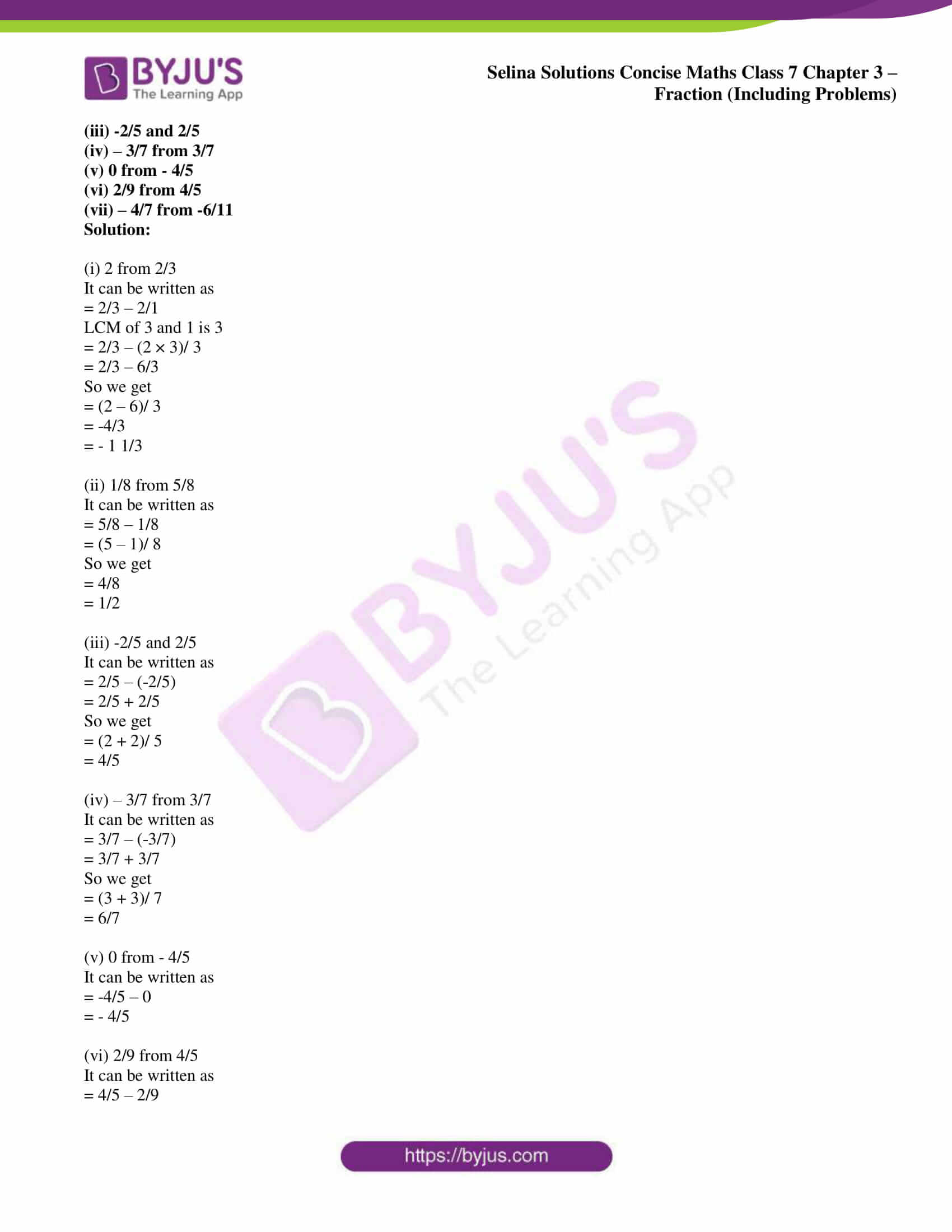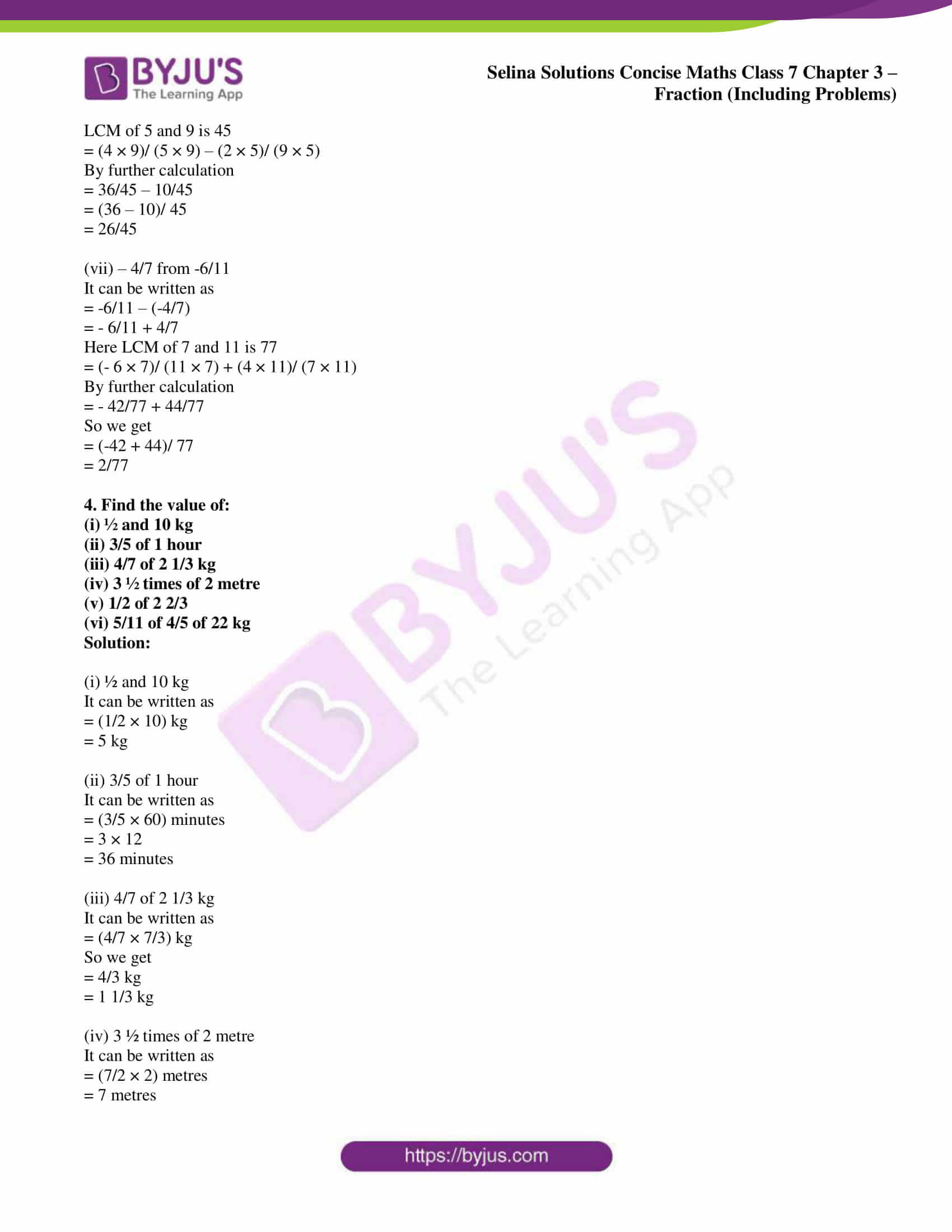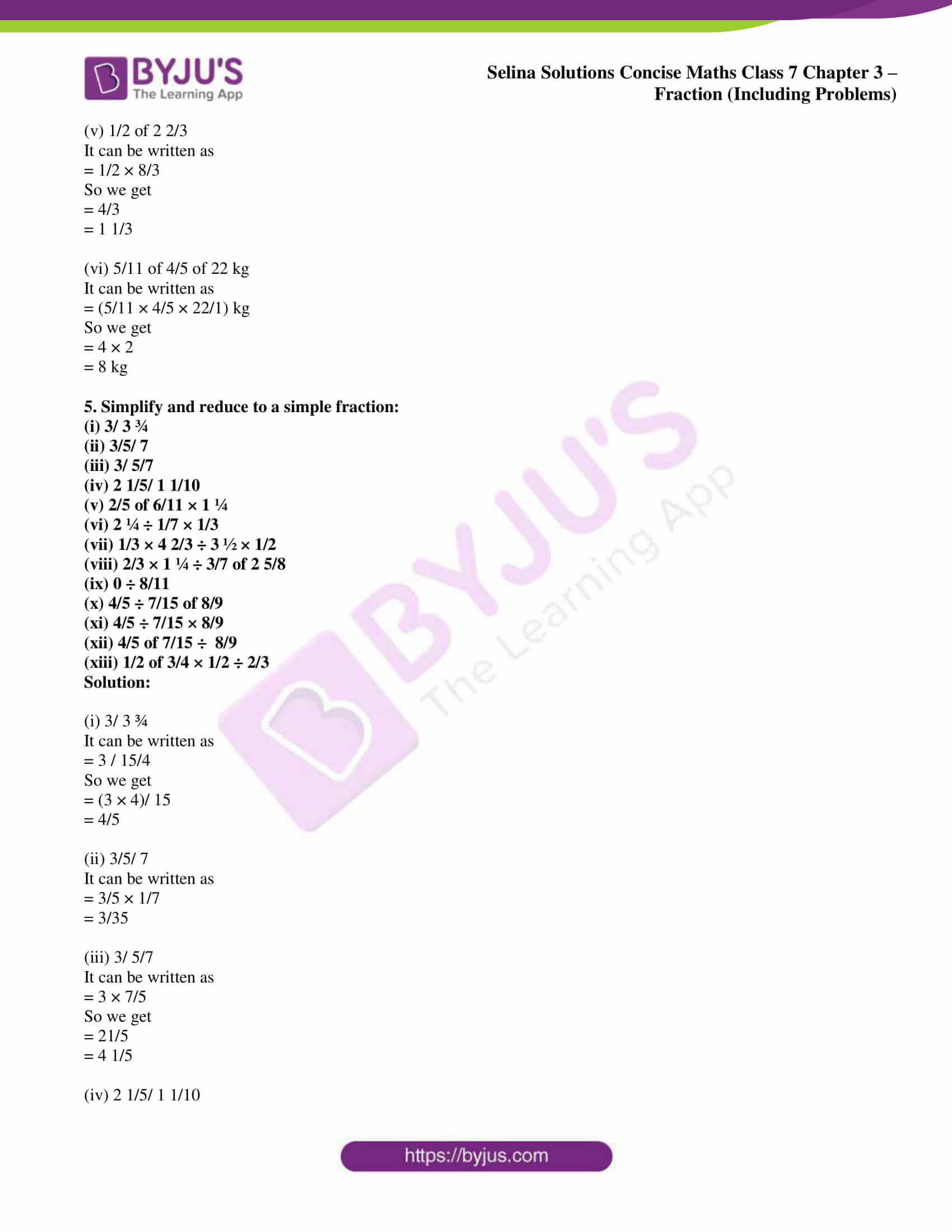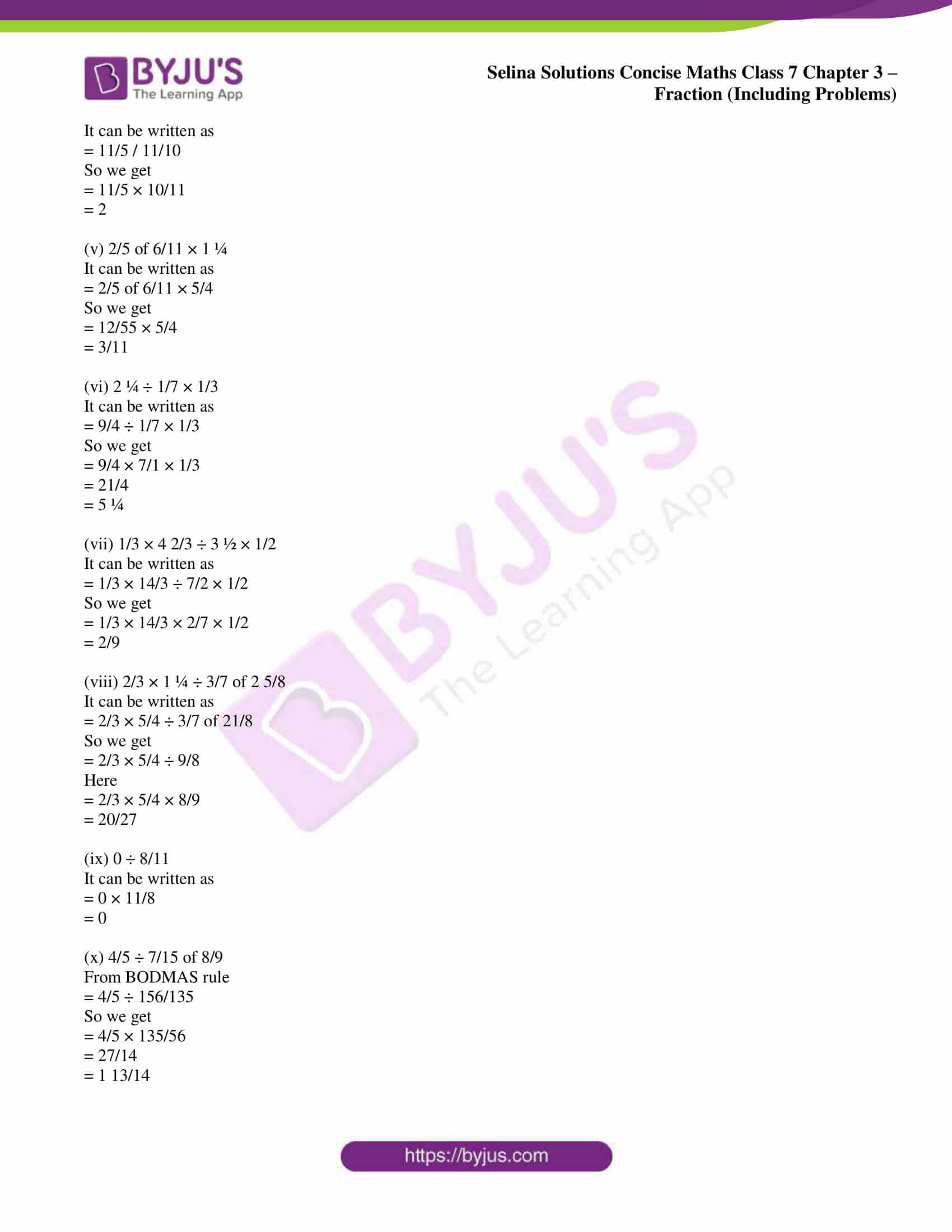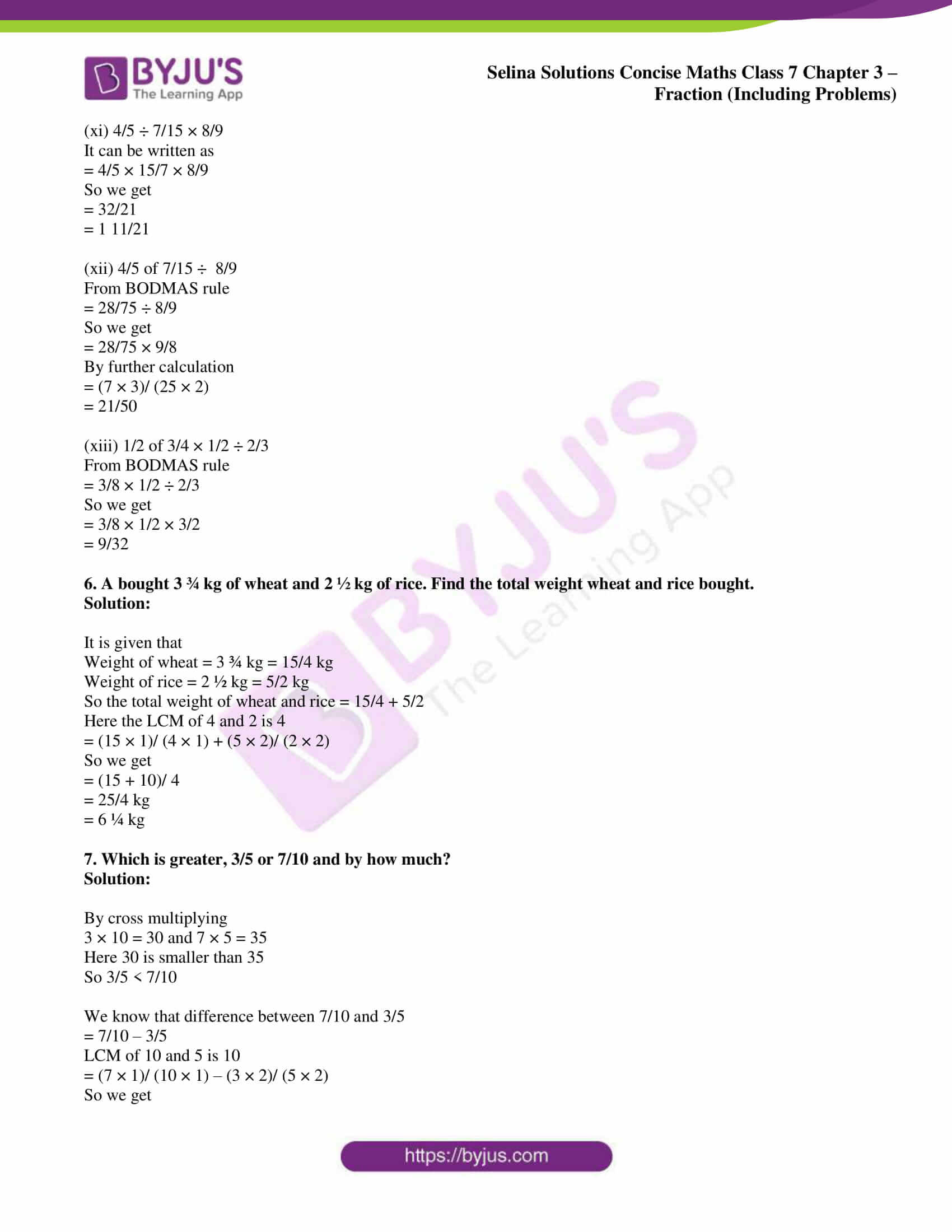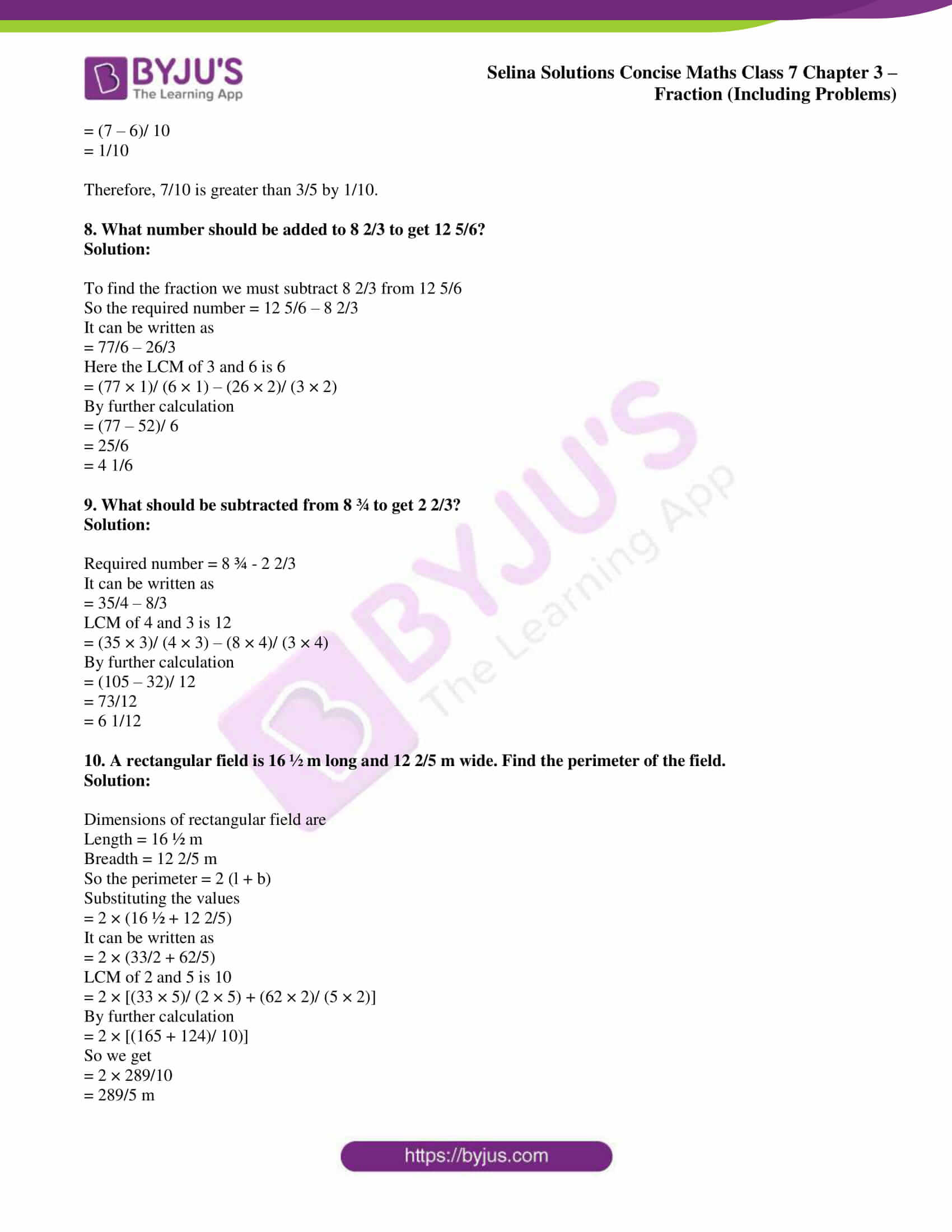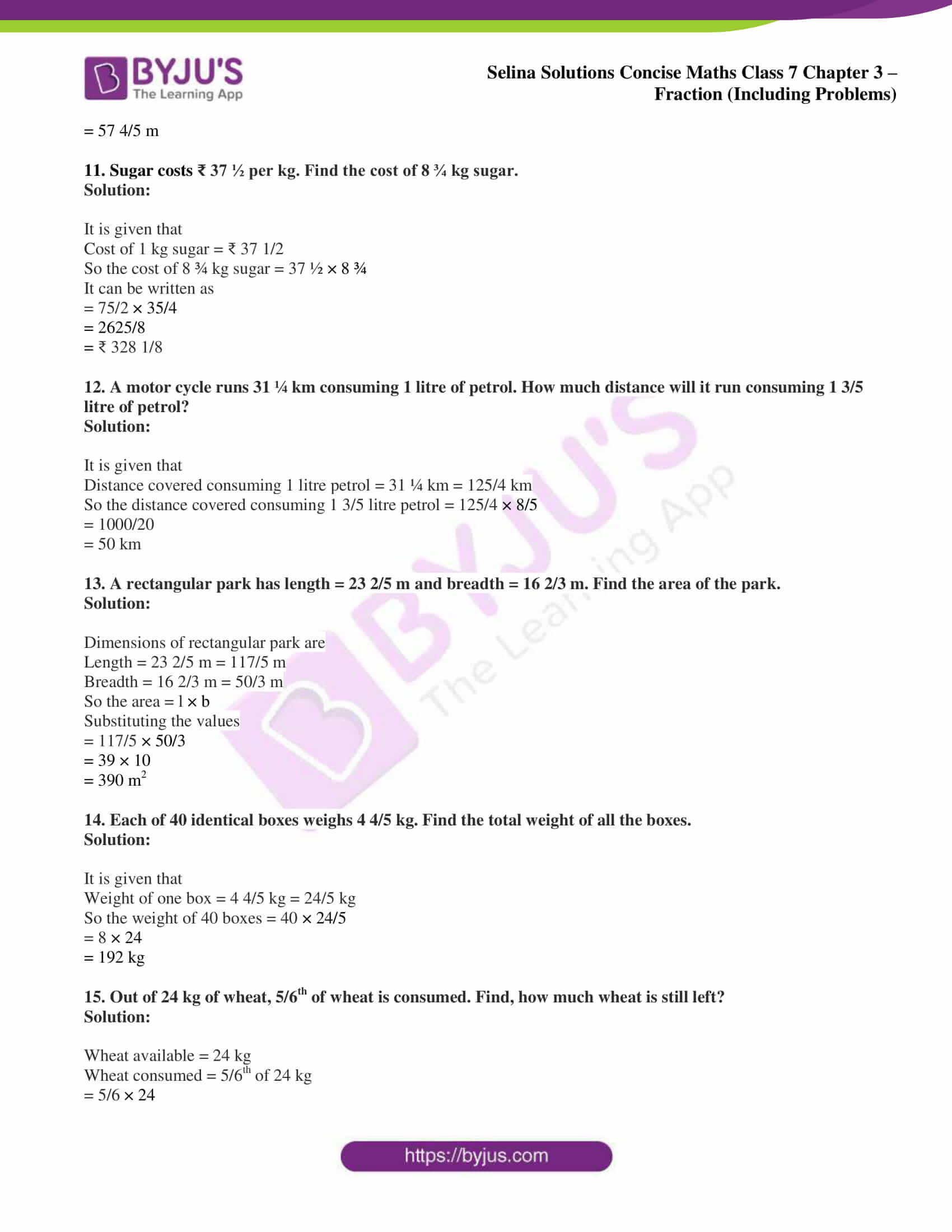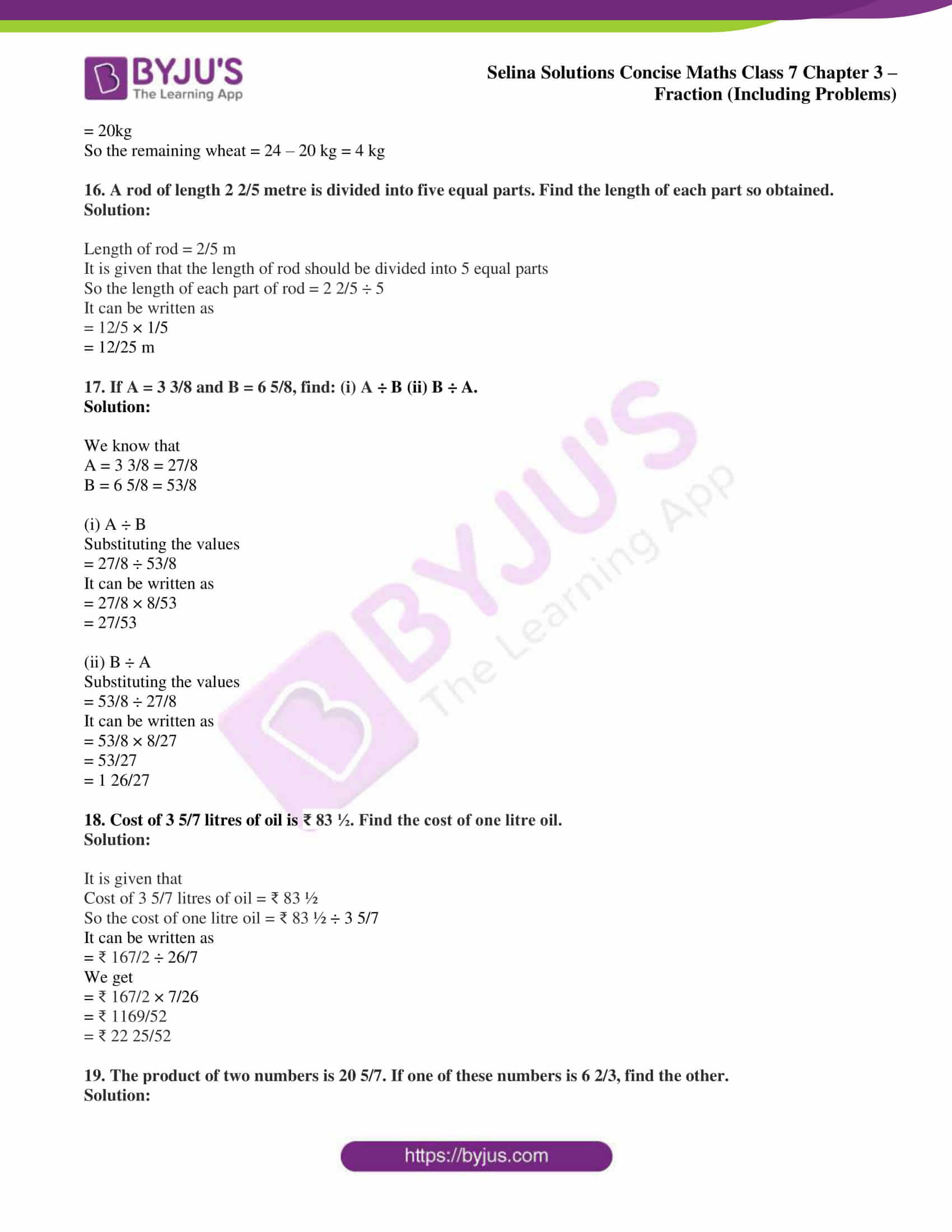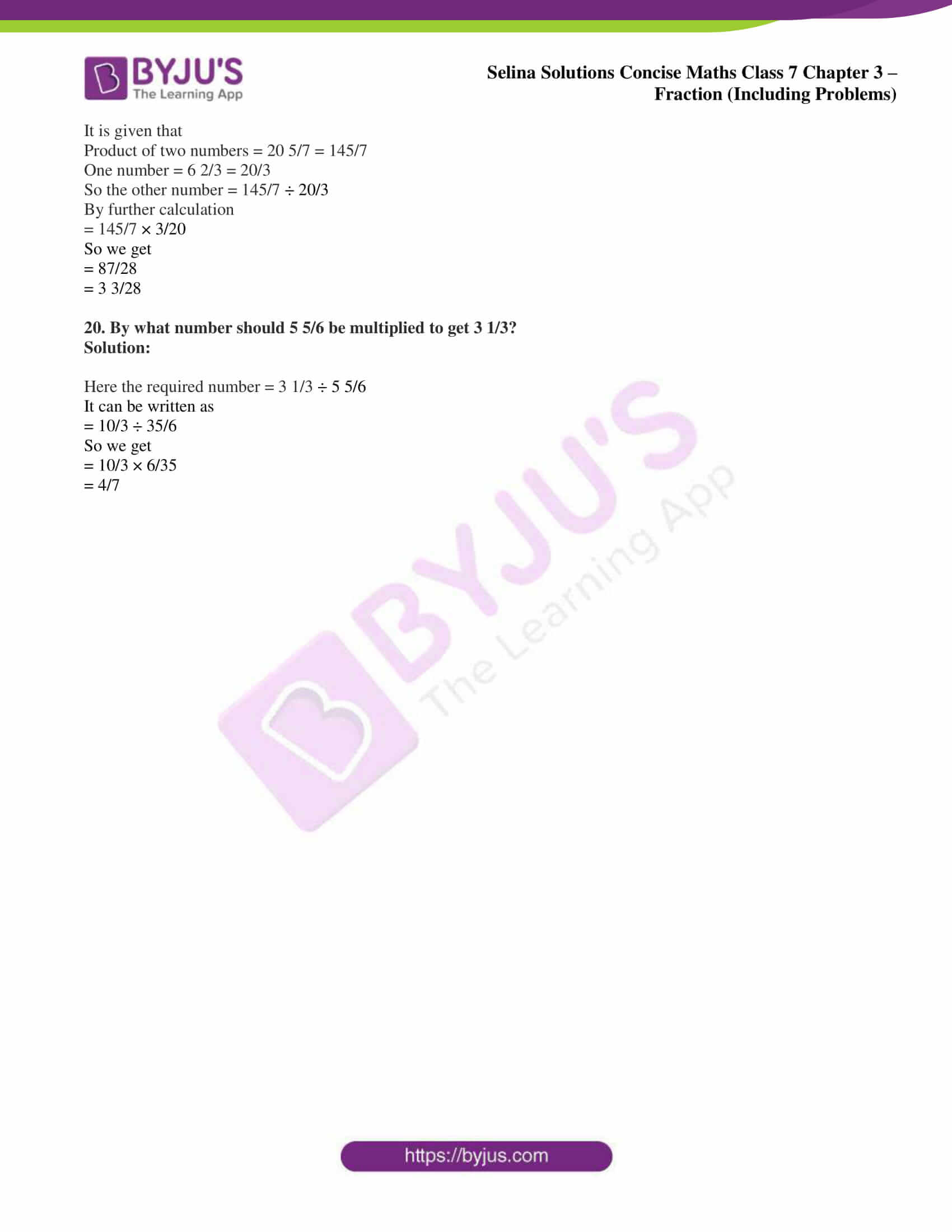### Access Selina Solutions Concise Maths Class 7 Chapter 3: Fraction (Including Problems) Exercise 3C

1. Reduce to a single fraction:

(i) 1/2 + 2/3

(ii) 3/5 – 1/10

(iii) 2/3 – 1/6

(iv) 1 1/3 + 2 1/4

(v) 1/4 + 5/6 – 1/12

(vi) 2/3 – 3/5 + 3 – 1/5

(vii) 2/3 – 1/5 + 1/10

(viii) 2 1/2 + 2 1/3 – 1 1/4

(ix) 2 5/8 – 2 1/6 + 4 3/4

Solution:

(i) 1/2 + 2/3

Here the LCM of 2 and 3 is 6

= (1 × 3)/ (2 × 3) + (2 × 2)/ (3 × 2)

By further calculation

= 3/6 + 4/6

= (3 + 4)/ 6

So we get

= 7/6

= 1 1/6

(ii) 3/5 – 1/10

Here the LCM of 5 and 10 is 10

= (3 × 2)/ (5 × 2) – 1/10

By further calculation

= 6/10 – 1/10

So we get

= (6 – 1)/ 10

= 5/10

= 1/2

(iii) 2/3 – 1/6

Here the LCM of 3 and 6 is 6

= (2 × 2)/ (3 × 2) – 1/6

By further calculation

= 4/6 – 1/6

So we get

= (4 – 1)/ 6

= 3/6

= 1/2

(iv) 1 1/3 + 2 1/4

It can be written as

= 4/3 + 9/4

Here LCM of 3 and 4 is 12

= (4 × 4)/ (3 × 4) + (9 × 3)/ (4 × 3)

By further calculation

= 16/12 + 27/12

So we get

= (16 + 27)/ 12

= 43/12

= 3 7/12

(v) 1/4 + 5/6 – 1/12

Here LCM of 4, 6 and 12 is 12

= (1 × 3)/ (4 × 3) + (5 × 2)/ (6 × 2) – 1/12

By further calculation

= 3/12 + 10/12 – 1/12

So we get

= (3 + 10 – 1)/ 12

= 12/12

= 1

(vi) 2/3 – 3/5 + 3 – 1/5

Here LCM of 3 and 5 is 15

= (2 × 5)/ (3 × 5) – (3 × 3)/ (5 × 3) + (3 × 15)/ 15 – (1 × 3)/ (5 × 3)

By further calculation

= 10/15 – 9/15 + 45/15 – 3/15

So we get

= (10 – 9 + 45 – 3)/ 15

= (55 – 12)/ 15

= 43/15

= 2 13/15

(vii) 2/3 – 1/5 + 1/10

Here the LCM of 3, 5 and 10 is 30

= (2 × 10)/ (3 × 10) – (1 × 6)/ (5 × 6) + (1 × 3)/ (10 × 3)

By further calculation

= 20/30 – 6/30 + 3/30

So we get

= (20 – 6 + 3)/ 30

= (23 – 6)/ 30

= 17/30

(viii) 2 1/2 + 2 1/3 – 1 ¼

It can be written as

= 5/2 + 7/3 – 5/4

Here the LCM of 2, 3 and 4 is 12

= (5 × 6)/ (2 × 6) + (7 × 4)/ (3 × 4) – (5 × 3)/ (4 × 3)

By further calculation

= 30/12 + 28/12 – 15/12

So we get

= (30 + 28 – 15)/ 12

= (58 – 15)/ 12

= 43/12

= 3 7/12

(ix) 2 5/8 – 2 1/6 + 4 3/4

It can be written as

= 21/8 – 13/6 + 19/4

Here the LCM of 8, 6 and 4 is 24

= (21 × 3)/ (8 × 3) – (13 × 4)/ (6 × 4) + (19 × 6)/ (4 × 6)

By further calculation

= 63/24 – 52/24 + 114/24

So we get

= (63 – 52 + 114)/ 24

= (177 – 52)/ 24

= 125/24

= 5 5/24

2. Simplify:

(i) 3/4 × 6

(ii) 2/3 × 15

(iii) 3/4 × 1/2

(iv) 9/12 × 4/7

(v) 45 × 2 1/3

(vi) 36 × 3 1/4

(vii) 2 ÷ 1/3

(viii) 3 ÷ 2/5

(ix) 1 ÷ 3/5

(x) 1/3 ÷ 1/4

(xi) -5/8 ÷ 3/4

(xii) 3 3/7 ÷ 1 1/14

(xiii) 3 ¾ × 1 1/5 × 20/21

Solution:

(i) 3/4 × 6

It can be written as

= 3/4 × 6/1

= (3 × 6)/ (4 × 1)

So we get

= 18/4

HCF of 18 and 4 is 2

Dividing both numerator and denominator by 2

= (18 ÷ 2)/ (4 ÷ 2)

= 9/2

= 4 1/2

(ii) 2/3 × 15

It can be written as

= 2/3 × 15/1

= (2 × 15)/ (3 × 1)

So we get

= 30/3

= 10

(iii) 3/4 × 1/2

It can be written as

= (3 × 1)/ (4 × 2)

= 3/8

(iv) 9/12 × 4/7

It can be written as

= (9 × 4)/ (12 × 7)

So we get

= 36/84

Here the HCF of 36 and 84 is 12

Dividing both numerator and denominator by 12

= (36 ÷ 12)/ (84 ÷ 12)

= 3/7

(v) 45 × 2 1/3

It can be written as

= 45/1 × 7/3

= (45 × 7)/ (1 × 3)

So we get

= 315/3

= 105

(vi) 36 × 3 1/4

It can be written as

= 36/1 × 13/4

= (36 × 13)/ (4 × 1)

So we get

= 468/4

= 117

(vii) 2 ÷ 1/3

It can be written as

= 2/1 × 3/1

= (2 × 3)/ (1 × 1)

So we get

= 6/1

= 6

(viii) 3 ÷ 2/5

It can be written as

= 3/1 × 5/2

= (3 × 5)/ (1 × 2)

So we get

= 15/2

= 7 ½

(ix) 1 ÷ 3/5

It can be written as

= 1 × 5/3

= (1 × 5)/ 3

So we get

= 5/3

= 1 2/3

(x) 1/3 ÷ 1/4

It can be written as

= 1/3 × 4/1

= (1 × 4)/ (3 × 1)

So we get

= 4/3

= 1 1/3

(xi) -5/8 ÷ 3/4

It can be written as

= -5/8 × 4/3

= (-5 × 4)/ (8 × 3)

So we get

= – 20/24

Here the HCF of 20 and 24 is 4

So by dividing both numerator and denominator by 4

= (-20 ÷ 4)/ (24 ÷ 4)

= -5/6

(xii) 3 3/7 ÷ 1 1/14

It can be written as

= (24 × 14)/ (7 × 15)

So we get

= 336/105

Here the HCF of 336 and 105 is 21

So by dividing both numerator and denominator by 21

= (336 ÷ 21)/ (105 ÷ 21)

= 16/5

= 3 1/5

(xiii) 3 ¾ × 1 1/5 × 20/21

It can be written as

= 15/4 × 6/5 × 20/21

= (15 × 6 × 20)/ (4 × 5 × 21)

So we get

= 1800/420

Here the HCF of 1800 and 420 is 60

So by dividing both numerator and denominator by 60

= (1800 ÷ 60)/ (420 ÷ 60)

= 30/7

= 4 2/7

3. Subtract:

(i) 2 from 2/3

(ii) 1/8 from 5/8

(iii) -2/5 and 2/5

(iv) – 3/7 from 3/7

(v) 0 from – 4/5

(vi) 2/9 from 4/5

(vii) – 4/7 from -6/11

Solution:

(i) 2 from 2/3

It can be written as

= 2/3 – 2/1

LCM of 3 and 1 is 3

= 2/3 – (2 × 3)/ 3

= 2/3 – 6/3

So we get

= (2 – 6)/ 3

= -4/3

= – 1 1/3

(ii) 1/8 from 5/8

It can be written as

= 5/8 – 1/8

= (5 – 1)/ 8

So we get

= 4/8

= 1/2

(iii) -2/5 and 2/5

It can be written as

= 2/5 – (-2/5)

= 2/5 + 2/5

So we get

= (2 + 2)/ 5

= 4/5

(iv) – 3/7 from 3/7

It can be written as

= 3/7 – (-3/7)

= 3/7 + 3/7

So we get

= (3 + 3)/ 7

= 6/7

(v) 0 from – 4/5

It can be written as

= -4/5 – 0

= – 4/5

(vi) 2/9 from 4/5

It can be written as

= 4/5 – 2/9

LCM of 5 and 9 is 45

= (4 × 9)/ (5 × 9) – (2 × 5)/ (9 × 5)

By further calculation

= 36/45 – 10/45

= (36 – 10)/ 45

= 26/45

(vii) – 4/7 from -6/11

It can be written as

= -6/11 – (-4/7)

= – 6/11 + 4/7

Here LCM of 7 and 11 is 77

= (- 6 × 7)/ (11 × 7) + (4 × 11)/ (7 × 11)

By further calculation

= – 42/77 + 44/77

So we get

= (-42 + 44)/ 77

= 2/77

4. Find the value of:

(i) ½ and 10 kg

(ii) 3/5 of 1 hour

(iii) 4/7 of 2 1/3 kg

(iv) 3 ½ times of 2 metre

(v) 1/2 of 2 2/3

(vi) 5/11 of 4/5 of 22 kg

Solution:

(i) ½ and 10 kg

It can be written as

= (1/2 × 10) kg

= 5 kg

(ii) 3/5 of 1 hour

It can be written as

= (3/5 × 60) minutes

= 3 × 12

= 36 minutes

(iii) 4/7 of 2 1/3 kg

It can be written as

= (4/7 × 7/3) kg

So we get

= 4/3 kg

= 1 1/3 kg

(iv) 3 ½ times of 2 metre

It can be written as

= (7/2 × 2) metres

= 7 metres

(v) 1/2 of 2 2/3

It can be written as

= 1/2 × 8/3

So we get

= 4/3

= 1 1/3

(vi) 5/11 of 4/5 of 22 kg

It can be written as

= (5/11 × 4/5 × 22/1) kg

So we get

= 4 × 2

= 8 kg

5. Simplify and reduce to a simple fraction:

(i) 3/ 3 ¾

(ii) 3/5/ 7

(iii) 3/ 5/7

(iv) 2 1/5/ 1 1/10

(v) 2/5 of 6/11 × 1 ¼

(vi) 2 ¼ ÷ 1/7 × 1/3

(vii) 1/3 × 4 2/3 ÷ 3 ½ × 1/2

(viii) 2/3 × 1 ¼ ÷ 3/7 of 2 5/8

(ix) 0 ÷ 8/11

(x) 4/5 ÷ 7/15 of 8/9

(xi) 4/5 ÷ 7/15 × 8/9

(xii) 4/5 of 7/15 ÷ 8/9

(xiii) 1/2 of 3/4 × 1/2 ÷ 2/3

Solution:

(i) 3/ 3 ¾

It can be written as

= 3 / 15/4

So we get

= (3 × 4)/ 15

= 4/5

(ii) 3/5/ 7

It can be written as

= 3/5 × 1/7

= 3/35

(iii) 3/ 5/7

It can be written as

= 3 × 7/5

So we get

= 21/5

= 4 1/5

(iv) 2 1/5/ 1 1/10

It can be written as

= 11/5 / 11/10

So we get

= 11/5 × 10/11

= 2

(v) 2/5 of 6/11 × 1 ¼

It can be written as

= 2/5 of 6/11 × 5/4

So we get

= 12/55 × 5/4

= 3/11

(vi) 2 ¼ ÷ 1/7 × 1/3

It can be written as

= 9/4 ÷ 1/7 × 1/3

So we get

= 9/4 × 7/1 × 1/3

= 21/4

= 5 ¼

(vii) 1/3 × 4 2/3 ÷ 3 ½ × 1/2

It can be written as

= 1/3 × 14/3 ÷ 7/2 × 1/2

So we get

= 1/3 × 14/3 × 2/7 × 1/2

= 2/9

(viii) 2/3 × 1 ¼ ÷ 3/7 of 2 5/8

It can be written as

= 2/3 × 5/4 ÷ 3/7 of 21/8

So we get

= 2/3 × 5/4 ÷ 9/8

Here

= 2/3 × 5/4 × 8/9

= 20/27

(ix) 0 ÷ 8/11

It can be written as

= 0 × 11/8

= 0

(x) 4/5 ÷ 7/15 of 8/9

From BODMAS rule

= 4/5 ÷ 156/135

So we get

= 4/5 × 135/56

= 27/14

= 1 13/14

(xi) 4/5 ÷ 7/15 × 8/9

It can be written as

= 4/5 × 15/7 × 8/9

So we get

= 32/21

= 1 11/21

(xii) 4/5 of 7/15 ÷ 8/9

From BODMAS rule

= 28/75 ÷ 8/9

So we get

= 28/75 × 9/8

By further calculation

= (7 × 3)/ (25 × 2)

= 21/50

(xiii) 1/2 of 3/4 × 1/2 ÷ 2/3

From BODMAS rule

= 3/8 × 1/2 ÷ 2/3

So we get

= 3/8 × 1/2 × 3/2

= 9/32

6. A bought 3 ¾ kg of wheat and 2 ½ kg of rice. Find the total weight wheat and rice bought.

Solution:

It is given that

Weight of wheat = 3 ¾ kg = 15/4 kg

Weight of rice = 2 ½ kg = 5/2 kg

So the total weight of wheat and rice = 15/4 + 5/2

Here the LCM of 4 and 2 is 4

= (15 × 1)/ (4 × 1) + (5 × 2)/ (2 × 2)

So we get

= (15 + 10)/ 4

= 25/4 kg

= 6 ¼ kg

7. Which is greater, 3/5 or 7/10 and by how much?

Solution:

By cross multiplying

3 × 10 = 30 and 7 × 5 = 35

Here 30 is smaller than 35

So 3/5 < 7/10

We know that difference between 7/10 and 3/5

= 7/10 – 3/5

LCM of 10 and 5 is 10

= (7 × 1)/ (10 × 1) – (3 × 2)/ (5 × 2)

So we get

= (7 – 6)/ 10

= 1/10

Therefore, 7/10 is greater than 3/5 by 1/10.

8. What number should be added to 8 2/3 to get 12 5/6?

Solution:

To find the fraction we must subtract 8 2/3 from 12 5/6

So the required number = 12 5/6 – 8 2/3

It can be written as

= 77/6 – 26/3

Here the LCM of 3 and 6 is 6

= (77 × 1)/ (6 × 1) – (26 × 2)/ (3 × 2)

By further calculation

= (77 – 52)/ 6

= 25/6

= 4 1/6

9. What should be subtracted from 8 ¾ to get 2 2/3?

Solution:

Required number = 8 ¾ – 2 2/3

It can be written as

= 35/4 – 8/3

LCM of 4 and 3 is 12

= (35 × 3)/ (4 × 3) – (8 × 4)/ (3 × 4)

By further calculation

= (105 – 32)/ 12

= 73/12

= 6 1/12

10. A rectangular field is 16 ½ m long and 12 2/5 m wide. Find the perimeter of the field.

Solution:

Dimensions of rectangular field are

Length = 16 ½ m

So the perimeter = 2 (l + b)

Substituting the values

= 2 × (16 ½ + 12 2/5)

It can be written as

= 2 × (33/2 + 62/5)

LCM of 2 and 5 is 10

= 2 × [(33 × 5)/ (2 × 5) + (62 × 2)/ (5 × 2)]

By further calculation

= 2 × [(165 + 124)/ 10)]
So we get

= 2 × 289/10

= 289/5 m

= 57 4/5 m

11. Sugar costs ₹ 37 ½ per kg. Find the cost of 8 ¾ kg sugar.

Solution:

It is given that

Cost of 1 kg sugar = ₹ 37 1/2

So the cost of 8 ¾ kg sugar = 37 ½ × 8 ¾

It can be written as

= 75/2 × 35/4

= 2625/8

= ₹ 328 1/8

12. A motor cycle runs 31 ¼ km consuming 1 litre of petrol. How much distance will it run consuming 1 3/5 litre of petrol?

Solution:

It is given that

Distance covered consuming 1 litre petrol = 31 ¼ km = 125/4 km

So the distance covered consuming 1 3/5 litre petrol = 125/4 × 8/5

= 1000/20

= 50 km

13. A rectangular park has length = 23 2/5 m and breadth = 16 2/3 m. Find the area of the park.

Solution:

Dimensions of rectangular park are

Length = 23 2/5 m = 117/5 m

Breadth = 16 2/3 m = 50/3 m

So the area = l × b

Substituting the values

= 117/5 × 50/3

= 39 × 10

= 390 m2

14. Each of 40 identical boxes weighs 4 4/5 kg. Find the total weight of all the boxes.

Solution:

It is given that

Weight of one box = 4 4/5 kg = 24/5 kg

So the weight of 40 boxes = 40 × 24/5

= 8 × 24

= 192 kg

15. Out of 24 kg of wheat, 5/6th of wheat is consumed. Find, how much wheat is still left?

Solution:

Wheat available = 24 kg

Wheat consumed = 5/6th of 24 kg

= 5/6 × 24

= 20kg

So the remaining wheat = 24 – 20 kg = 4 kg

16. A rod of length 2 2/5 metre is divided into five equal parts. Find the length of each part so obtained.

Solution:

Length of rod = 2/5 m

It is given that the length of rod should be divided into 5 equal parts

So the length of each part of rod = 2 2/5 ÷ 5

It can be written as

= 12/5 × 1/5

= 12/25 m

17. If A = 3 3/8 and B = 6 5/8, find: (i) A ÷ B (ii) B ÷ A.

Solution:

We know that

A = 3 3/8 = 27/8

B = 6 5/8 = 53/8

(i) A ÷ B

Substituting the values

= 27/8 ÷ 53/8

It can be written as

= 27/8 × 8/53

= 27/53

(ii) B ÷ A

Substituting the values

= 53/8 ÷ 27/8

It can be written as

= 53/8 × 8/27

= 53/27

= 1 26/27

18. Cost of 3 5/7 litres of oil is ₹ 83 ½. Find the cost of one litre oil.

Solution:

It is given that

Cost of 3 5/7 litres of oil = ₹ 83 ½

So the cost of one litre oil = ₹ 83 ½ ÷ 3 5/7

It can be written as

= ₹ 167/2 ÷ 26/7

We get

= ₹ 167/2 × 7/26

= ₹ 1169/52

= ₹ 22 25/52

19. The product of two numbers is 20 5/7. If one of these numbers is 6 2/3, find the other.

Solution:

It is given that

Product of two numbers = 20 5/7 = 145/7

One number = 6 2/3 = 20/3

So the other number = 145/7 ÷ 20/3

By further calculation

= 145/7 × 3/20

So we get

= 87/28

= 3 3/28

20. By what number should 5 5/6 be multiplied to get 3 1/3?

Solution:

Here the required number = 3 1/3 ÷ 5 5/6

It can be written as

= 10/3 ÷ 35/6

So we get

= 10/3 × 6/35

= 4/7